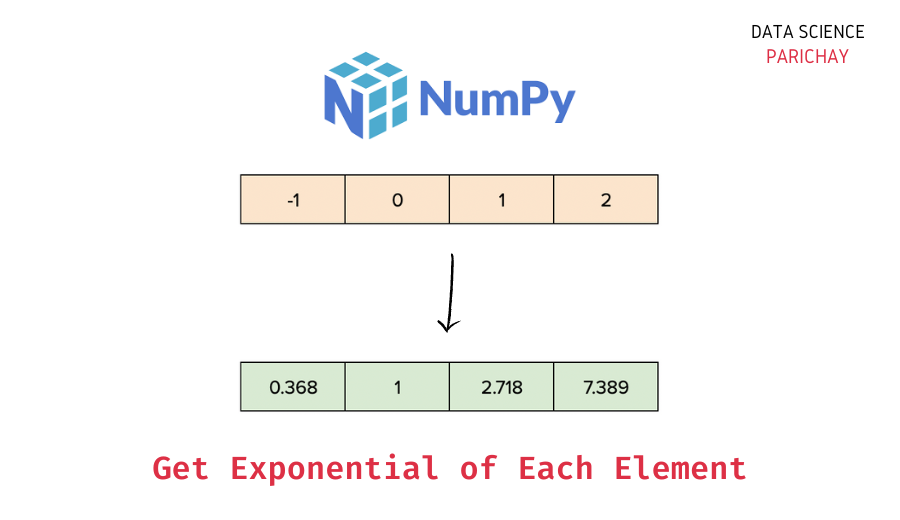# Get the Exponential of Each Element in Numpy Array

The Numpy library in Python comes with a number of built-in functions to perform common mathematical operations on arrays. In this tutorial, we will look at one such function that helps us get the element-wise exponential of a Numpy array with the help of some examples.

## How to get the exponential in Numpy?

You can use the `numpy.exp()` function to get the exponential of all elements in a Numpy array. Pass the array as an argument.

The following is the syntax –

`numpy.exp(ar)`

It returns an array containing the exponential value of each element in the passed array.

Let’s now look at a step-by-step example of using the `numpy.exp()` function.

### Step 1 – Create a Numpy array

First, we will create a Numpy array that we will use throughout this tutorial.

```import numpy as np

# create numpy array
ar = np.array([-10, -1, 0, 1, 2, 10])
# display the array
print(ar)```

Output:

`[-10  -1   0   1   2  10]`

Here, we used the `numpy.array()` function to create a Numpy array containing some numbers. You can see that the array contains both positive and negative integer values (along with a zero).

📚 Data Science Programs By Skill Level

Introductory

Intermediate ⭐⭐⭐

🔎 Find Data Science Programs 👨‍💻 111,889 already enrolled

Disclaimer: Data Science Parichay is reader supported. When you purchase a course through a link on this site, we may earn a small commission at no additional cost to you. Earned commissions help support this website and its team of writers.

### Step 2 – Get the exponential using `numpy.exp()`

To get the exponential of each element in a Numpy array, pass the array as an argument to the `numpy.exp()` function.

Let’s get the exponential for the array created above.

```# get the exponential of each element
np.exp(ar)```

Output:

```array([4.53999298e-05, 3.67879441e-01, 1.00000000e+00, 2.71828183e+00,
7.38905610e+00, 2.20264658e+04])```

We get a Numpy array with the exponential value of each element in the array `ar`.

The `numpy.exp()` function works similarly on higher-dimensional arrays. For example, let’s apply this function to a 2D array of some numbers.

```# create 2D numpy array
ar = np.array([[1, 0, 1],
[0, 10, 2],
[-10, 0, 1]])
# get the exponential of each element
np.exp(ar)```

Output:

```array([[2.71828183e+00, 1.00000000e+00, 2.71828183e+00],
[1.00000000e+00, 2.20264658e+04, 7.38905610e+00],
[4.53999298e-05, 1.00000000e+00, 2.71828183e+00]])```

You can see that we get the exponential value of each element in the 2D array.

For more on the `numpy.exp()` function, refer to its documentation.

You might also be interested in –

•